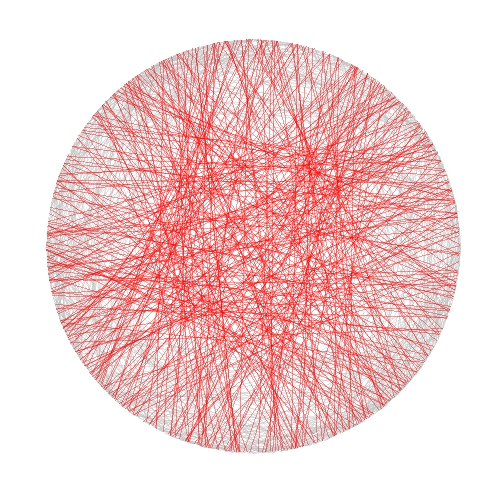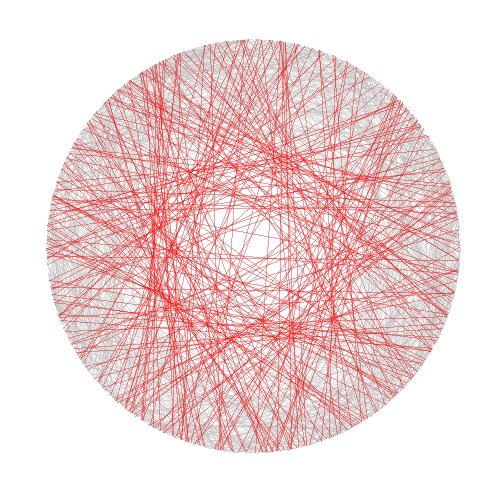# Bertrand or (The Importance of Defining Problems Properly)

We better keep an eye on this one: she is tricky (Michael Banks, talking about Mary Poppins)

Professor Bertrand teaches Simulation and someday, ask his students:

Given a circumference, what is the probability that a chord chosen at random is longer than a side of the equilateral triangle inscribed in the circle?

Since they must reach the answer through simulation, very approximate solutions are welcome.

Some students choose chords as the line between two random points on the circumference and conclude that the asked probability is around 1/3. This is the plot of one of their simulations, where 1000 random chords are chosen according this method and those longer than the side of the equilateral triangle are red coloured (smalller in grey):Some others choose a random radius and a random point in it. The chord then is the perpendicular through this point. They calculate that the asked probability is around 1/2:And some others choose a random point inside the circle and define the chord as the only one with this point as midpoint. For them, the asked probability is around 1/4:Who is right? Professor Bertrand knows that everybody is. In fact, his main purpose was to show how important is to define problems properly. Actually, he used this to give an unforgettable lesson to his students.

```library(ggplot2)
n=1000
opt=theme(legend.position="none",
panel.background = element_rect(fill="white"),
panel.grid = element_blank(),
axis.ticks=element_blank(),
axis.title=element_blank(),
axis.text =element_blank())
#First approach
angle=runif(2*n, min = 0, max = 2*pi)
pt1=data.frame(x=cos(angle), y=sin(angle))
df1=cbind(pt1[1:n,], pt1[((n+1):(2*n)),])
colnames(df1)=c("x1", "y1", "x2", "y2")
df1\$length=sqrt((df1\$x1-df1\$x2)^2+(df1\$y1-df1\$y2)^2)
p1=ggplot(df1) + geom_segment(aes(x = x1, y = y1, xend = x2, yend = y2, colour=length>sqrt(3)), alpha=.4, lwd=.6)+
scale_colour_manual(values = c("gray75", "red"))+opt
#Second approach
angle=2*pi*runif(n)
pt2=data.frame(aa=cos(angle), bb=sin(angle))
pt2\$x0=pt2\$aa*runif(n)
pt2\$y0=pt2\$x0*(pt2\$bb/pt2\$aa)
pt2\$a=1+(pt2\$x0^2/pt2\$y0^2)
pt2\$b=-2*(pt2\$x0/pt2\$y0)*(pt2\$y0+(pt2\$x0^2/pt2\$y0))
pt2\$c=(pt2\$y0+(pt2\$x0^2/pt2\$y0))^2-1
pt2\$x1=(-pt2\$b+sqrt(pt2\$b^2-4*pt2\$a*pt2\$c))/(2*pt2\$a)
pt2\$y1=-pt2\$x0/pt2\$y0*pt2\$x1+(pt2\$y0+(pt2\$x0^2/pt2\$y0))
pt2\$x2=(-pt2\$b-sqrt(pt2\$b^2-4*pt2\$a*pt2\$c))/(2*pt2\$a)
pt2\$y2=-pt2\$x0/pt2\$y0*pt2\$x2+(pt2\$y0+(pt2\$x0^2/pt2\$y0))
df2=pt2[,c(8:11)]
df2\$length=sqrt((df2\$x1-df2\$x2)^2+(df2\$y1-df2\$y2)^2)
p2=ggplot(df2) + geom_segment(aes(x = x1, y = y1, xend = x2, yend = y2, colour=length>sqrt(3)), alpha=.4, lwd=.6)+
scale_colour_manual(values = c("gray75", "red"))+opt
#Third approach
angle=2*pi*runif(n)
pt3\$a=1+(pt3\$x0^2/pt3\$y0^2)
pt3\$b=-2*(pt3\$x0/pt3\$y0)*(pt3\$y0+(pt3\$x0^2/pt3\$y0))
pt3\$c=(pt3\$y0+(pt3\$x0^2/pt3\$y0))^2-1
pt3\$x1=(-pt3\$b+sqrt(pt3\$b^2-4*pt3\$a*pt3\$c))/(2*pt3\$a)
pt3\$y1=-pt3\$x0/pt3\$y0*pt3\$x1+(pt3\$y0+(pt3\$x0^2/pt3\$y0))
pt3\$x2=(-pt3\$b-sqrt(pt3\$b^2-4*pt3\$a*pt3\$c))/(2*pt3\$a)
pt3\$y2=-pt3\$x0/pt3\$y0*pt3\$x2+(pt3\$y0+(pt3\$x0^2/pt3\$y0))
df3=pt3[,c(6:9)]
df3\$length=sqrt((df3\$x1-df3\$x2)^2+(df3\$y1-df3\$y2)^2)
p3=ggplot(df3) + geom_segment(aes(x = x1, y = y1, xend = x2, yend = y2, colour=length>sqrt(3)), alpha=.4, lwd=.6)+scale_colour_manual(values = c("gray75", "red"))+opt
```

## 1 thought on “Bertrand or (The Importance of Defining Problems Properly)”

1.statisticsblogdotcom says:

Nice demo. BTW E. T. Jaynes does a nice job of showing how certain assumptions about invariance lead to a better defined problem.